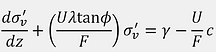## Corrigendum

Page 333, last paragraph, first sentence - 'delection' should say 'deflection'.

Page 418, Figure 12.2 - Legend on figure should refer to Worked Example 12.1 and 12.3 (not 11.1 and 11.3).

Page 490, Worked Example 13.2 - The sagging zone resultant diagonal strain of 0.039% should result in Damage Category 0 - 'negligible' (not Damage Category 1 - 'very slight').

Page 522, Equation A.14 - The 'exp' should not be there and the equation should be as shown below. This has no impact on any other equations. Thankyou to Rui Pontes for finding this one.

Page 528, Equation A.34 - Since the right hand side of this equation is just a substitution for the average shear stress using Equation A.31, there should be a term for cohesion c and Equation A.34 should look like this:

Page 531 - The value for Gs should be 2665.24 kN. This is a typo and the other values and Figure A.7 are correct. Thankyou Berk Demir for spotting this and the previous error.